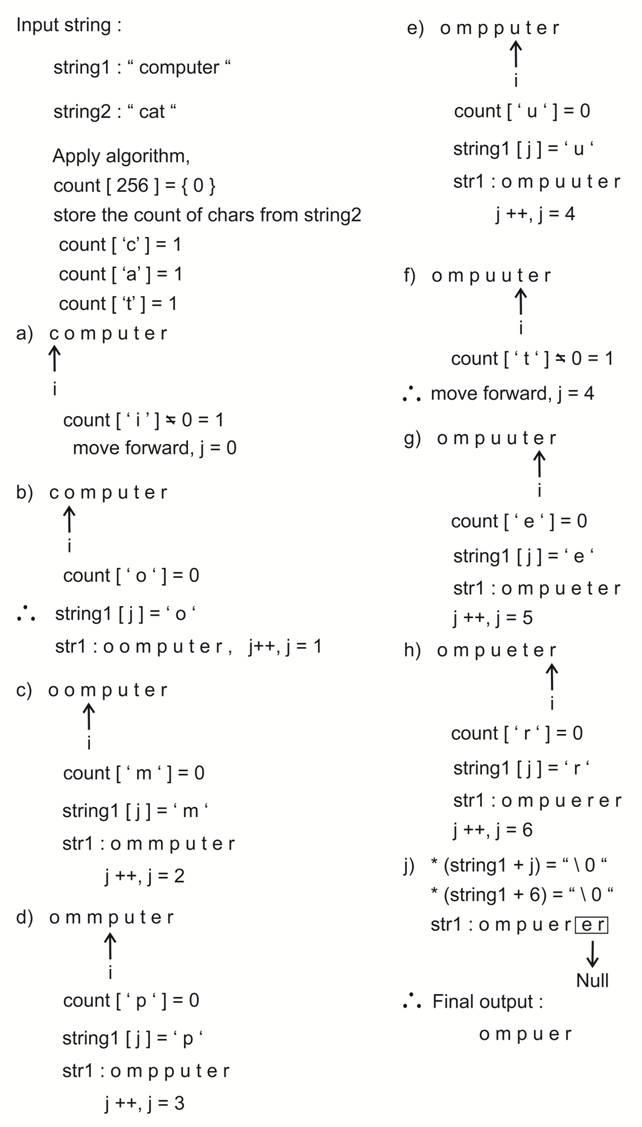# Remove characters from first string which are in second

StringViews 3759

## For the given input strings, remove the characters from the first string which are present in second string. (with case sensitivity)

### Example

a) Input string1 : computer
Input string2 : cat

Output : ompuer
After removing characters from string2 (c, a, t) from string1 we get ompuer

b) Input string1 : occurrence
Input string2 : car
Output : ouene

After removing characters from string2 (c, a, r) from string1 we get ouene

Time complexity : O(m + n), m and n are length of strings

## Algorithm

a. Get count array from the second string which stores the count of chars from the second string.

b. Check in the input string if it contains characters from count array with frequency > 0 if yes skip it and copy remaining char into input string.

c. After getting this add \0 to remove any extra characters after output string
(Null termination).

Note : \0 means Null ASCII value 0)

### Algorithm working## C++ Program

```#include <bits/stdc++.h>

using namespace std;
#define ASCII_SIZE 256

//Remove characters from string1 which are in string2
char *RemoveChars(char *string1, char *string2)
{
//Count array stores the count of chars from string2
int *count = (int *)calloc(sizeof(int), ASCII_SIZE);
for(int i = 0; *(string2+i);  i++)
{
count[*(string2+i)]++;
}
int i  = 0, j = 0;
while(*(string1 + i))
{
char temp = *(string1 + i);
//If count of charcter is zero add to output
if(count[temp] == 0)
{
*(string1 + j) = *(string1 + i);
j++;
}
i++;
}
//Null termination
//removing extra characters
*(string1+j) = '\0';

return string1;
}

//Main function to test above function
int main()
{
//string1
char string1[]  = "computer";
//string2
char string2[]  = "programming";
cout<<"Input strings:\n";
cout<<"string1: ";
for (int i = 0; i < strlen(string1); ++i)
{
cout<<string1[i];
}
cout<<"\nstring2: ";
for (int i = 0; i < strlen(string2); ++i)
{
cout<<string2[i];
}
//print output string
cout<<"\nOutput: ";
cout<<RemoveChars(string1, string2);
return 0;
}```

Translate »